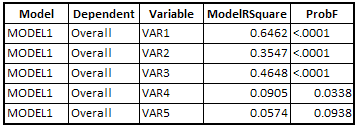# Selecting the Best Linear Regression Model

When you have a good number of variables in your regression model, it is very important to select the important variables so that the model would not overfit or underfit.

Method 1 : Univariate Analysis

The initial step is to check each independent variable with dependent variable. It is to eliminate some independent variables which are not related to dependent variable at all. We can check their individual model R-square and p-value of their coefficient to test whether coefficient is significantly different from zero.

Many researchers do not rely on univariate analysis to select important predictors as the final model which is a combination of multiple independent variables create different association altogether. Hence, it is important to select higher level of significance as standard 5% level. It is generally recommended to select 0.35 as criteria.

Suppose you have 1000 predictors in your regression model. It is memory intensive to run regression model 1000 times to produce R2 of each variable. In SAS, there is a optimized way to accomplish it.
ODS OUTPUT EntryStatistics = TEST;
Model Overall = VAR1 - VAR5/ SELECTION = STEPWISE MAXSTEP = 1 DETAILS;
run;
The above program produces univariate model for each of the 5 predictors. The output is shown in the image below -Univariate Regression Model Output
We can sort variables based on ModelRsquare value by descending order. Also put criteria on ProbF <=0.35.

Method 2 : Stepwise Selection Algorithm

The STEPWISE selection algorithm is a combination of backward and forward selection. In a forward stepwise regression, the variable which would add the largest increment to R2 (i.e. the variable which would have the largest semipartial correlation) is added next (provided it is statistically significant). In a backwards stepwise regression, the variable which would produce the smallest decrease in R2 (i.e. the variable with the smallest semipartial correlation) is dropped next (provided it is not statistically significant).
MODEL OVERALL = VAR1 - VAR5 / SELECTION = STEPWISE SLENTRY=0.3 SLSTAY=0.35;
RUN;
Note : SLENTRY=0.3 implies a variable would be added into the model if p-value <=0.3, and SLSTAY=0.35 means variable would be stayed in the model only if p-value <=0.35.

Method 3 : Automated Model Selection

There are two important metrics that helps evaluate the model - Adjusted R-Square and Mallows' Cp Statistics.

It penalizes the model for inclusion of each additional variable. Adjusted R-square would increase only if the variable included in the model is significant. The model with the larger adjusted R-square value is considered to be the better model.

SAS Code : Automatic selection of Best Model
model weight = height var1 var2 var3/ selection = adjrsq best = 1;
run;
run;

Mallows' Cp Statistic

It helps detect model biasness, which refers to either underfitting the model or overfitting the model.
Mallows Cp = (SSE/MSE) – (n – 2p)
where SSE is Sum of Squared Error and MSE is Mean Squared Error with all independent variables in model and p is for the number of estimates in model (i.e. number of independent variables plus intercept).

Rules

Look for models where Cp is less than or equal to p, which is the number of independent variables plus intercept.

A final model should be selected based on the following two criterias -

First Step : Models in which number of variables where Cp is less than or equal to p

Second Step : Select model in which fewest parameters exist.
Suppose two models have Cp less than or equal to p. First Model - 5 Variables, Second Model - 6 Variables. We should select first model as it contains fewer parameters.

Important Note :

To select the best model for parameter estimation, you should use Hocking's criterion for Cp.

For parameter estimation, Hocking recommends a model where Cp<=2p – pfull +1, where p is the number of parameters in the model, including the intercept. pfull - total number of parameters (initial variable list) in the model.

To select the best model for prediction, you should use Mallows' criterion for Cp.

SAS Code : Automatic selection of Best Model
proc reg data= class outest=outcp;
model weight = height var1 var2 var3/ selection = cp best 1;
run;
proc print data=outcp;
run;
Related Posts
Share1.